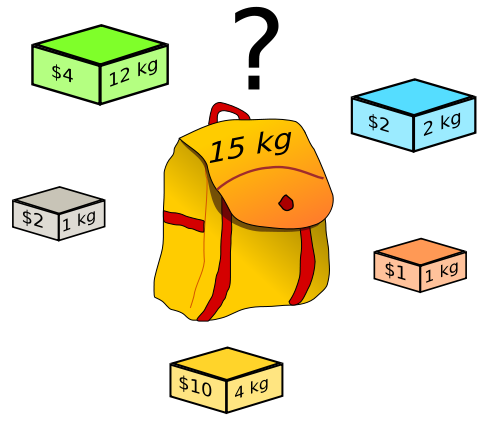# Greedy Algorithm

## 1 Greedy algorithm简介

A greedy algorithm is an algorithm that follows the problem solving heuristic of making the locally optimal choice at each stage with the hope of finding a global optimum.

Introduction to Algorithms, 3rd Edition. Chapter 16 Greedy Algorithms
"Greedy Exchange": a technique used in proving the correctness of greedy algorithms
Guide to Greedy Algorithms

## 2 实例：活动选择问题

$\begin{array}{c|ccccccccccc} i & 1 & 2 & 3 & 4 & 5 & 6 & 7 & 8 & 9 & 10 & 11 \\ \hline s_{i} & 1 & 3 & 0 & 5 & 3 & 5 & 6 & 8 & 8 & 2 & 12 \\ f_{i} & 4 & 5 & 6 & 7 & 9 & 9 & 10 & 11 & 12 & 14 & 16 \end{array}$

### 2.2 解法2：贪心算法

+-----+--------+--------+   +-------+
|     |        |        |   |       |
1--2--3--4--5--6--7--8--9--10--11--12
|  |        |     |             |   |
+--+        +-----+             +---+


#### 2.2.1 活动选择问题贪心算法实现

/* 贪心算法解决活动选择问题 */
#include<stdio.h>

void greedy_activity(int s[], int f[], int n) {
int i = 1;                                       // 由于约定f按单调递增的顺序排列，所以可以总是选择a1。
printf("The selected activities are: a%d ", i);
int j;
for (j=1; j<n; j++) {
if (s[j] >= f[i]) {
printf("a%d ", j+1);
i = j;
}
}
printf("\n");
}

int main() {
int s[] =  {1, 3, 0, 5, 3, 5,  6,  8,  8,  2, 12};   // 活动开始时间
int f[] =  {4, 5, 6, 7, 9, 9, 10, 11, 12, 14, 16};   // 活动结束时间
int n = sizeof(s)/sizeof(s);

greedy_activity(s, f, n);

return 0;
}


## 5 实例：背包问题Figure 1: 背包问题（摘自其 Wikipedia

### 5.1 0-1 knapsack problem（不能用贪心算法）

$m[i, w] = \begin{cases} 0 & \text{if} \; i=0 \; \text{or} \; w=0 \\ m[i-1, w] & \text{if} \; w_i > w \\ \max(m[i-1,w], m[i-1,w-w_i] + v_i) & \text{if} \; w_i \le w \\ \end{cases}$

// pseudo code for 0-1 knapsack problem

// Input:
// Values (stored in array v)
// Weights (stored in array w)
// Number of distinct items (n)
// Knapsack capacity (W)

for j from 0 to W do:
m[0, j] := 0

for i from 1 to n do:
for j from 0 to W do:
if w[i] > j then:
m[i, j] := m[i-1, j]
else:
m[i, j] := max(m[i-1, j], m[i-1, j-w[i]] + v[i])


### 5.2 fractional knapsack problem（贪心算法）

Created: <2011-09-03 Sat 00:00>

Last updated: <2017-12-16 Sat 17:37>

Creator: Emacs 25.3.1 (Org mode 9.1.4)